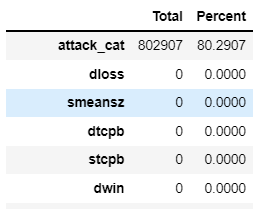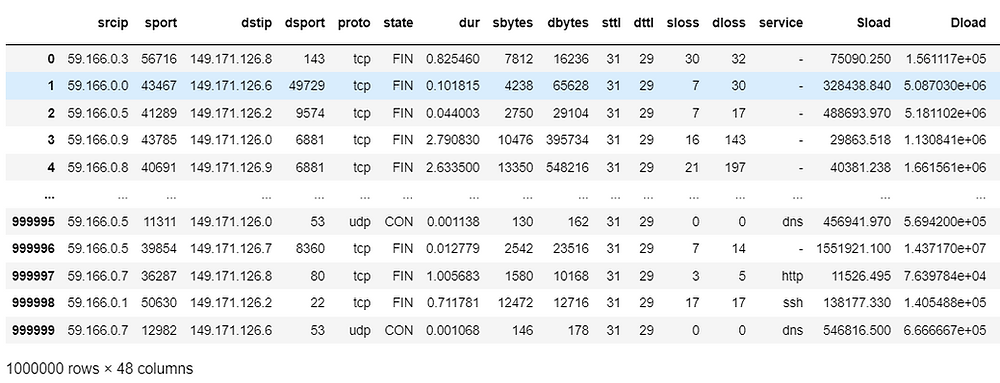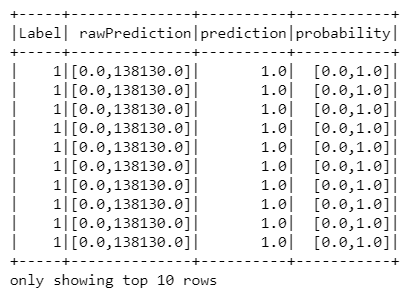Search

# Implement Multi-Classifier and And Binary Classifier Using Big Data PySpark

### Abstract

In artificial intelligence, machine learning is a method of acquiring knowledge that may be used to make intelligent judgments. There are many benefits to using big data for scientific research and value generation. This report introduces machine learning methods, Big Data technology, and machine learning applications in Big Data. There is a discussion of the challenges of machine learning applications with big data. Also highlighted are several new machine learning methodologies and technological advancements in Big Data. The Spark Python API (PySpark) exposes the Spark programming model to Python. Apache® Spark™ is an open source and is one of the most popular Big Data frameworks for scaling up your tasks in a cluster. It was developed to utilize distributed, in-memory data structures to improve data processing speeds. Apache Spark! The top technology companies like Google, Facebook, Netflix, Airbnb, Amazon, NASA, and more are all using Spark to solve their big data problems!

### Introduction

Machine learning is an important area of artificial intelligence. The goal of machine learning is to discover knowledge and make intelligent decisions. Machine learning algorithms can be classified into which are classified into supervised, unsupervised, and semi-supervised. When it comes to big data, scaling machine learning algorithms are needed (Chen and Zhang, 2014; Tarwani et al., 2015), Another classification of machine learning based on the output of the machine learning system includes classification, regression, clustering, and density estimation, etc. machine-learning approaches include decision tree learning, association rule learning, artificial neural networks, support vector machines (SVM), clustering, Bayesian networks, and genetic algorithms, etc.

### Initiate and Configure Spark

```!apt-get install openjdk-8-jdk-headless -qq > /dev/null
!pip install -q findspark
import os
os.environ["JAVA_HOME"] = "C:\work\Java\jre1.8.0_301"
import findspark
findspark.init()
​
from pyspark import SparkContext
from pyspark.sql import SQLContext
sc = SparkContext.getOrCreate()
sqlContext = SQLContext(sc)```

```import pandas as pd
#converting to normal dataframe
df=pd.DataFrame(data.take(1000000), columns=collist)

Output:```# checking missing data in stack data
total = df.isnull().sum().sort_values(ascending = False)
percent = (df.isnull().sum()/df.isnull().count()*100).sort_values(ascending = False)
missing_stack_data  = pd.concat([total, percent], axis=1, keys=['Total', 'Percent'])
missing_stack_data```

Output:...

...

```#Droping Null Values and Label column (as it work as the row number)
df_2=df.dropna()
df_2=df_2.drop(columns='Label')
df_2```

Output:```df_1 = df.drop(columns='attack_cat')
df_1```

Output:```#Extracting the categorical columns
cat_col = [key for key in dict(df_1.dtypes)
if dict(df_1.dtypes)[key] in ['object'] ] # Categorical Varible```
`cat_col`

Output:

`['srcip', 'dstip', 'proto', 'state', 'service']`

```#Extracting the numerical columns
num_col=list(df_1.select_dtypes(exclude=['object']).columns)```

```from pyspark.ml.feature import OneHotEncoder
from pyspark.ml.feature import StringIndexer, VectorAssembler```

The procedure incorporates Category Indexing, One-Hot Encoding, and VectorAssembler, a feature converter that merges many columns into a vector column.

```stages = []
for categoricalCol in cat_col:
stringIndexer = StringIndexer(inputCol = categoricalCol, outputCol = categoricalCol + 'Index')
encoder = OneHotEncoder(inputCols=[stringIndexer.getOutputCol()], outputCols=[categoricalCol + "classVec"])
stages += [stringIndexer, encoder]
assemblerInputs = [c + "classVec" for c in cat_col] + num_col
assembler = VectorAssembler(inputCols=assemblerInputs, outputCol="features")
stages += [assembler]```

`stages`

output:

```[StringIndexer_50fb84705796,
OneHotEncoder_16529dc18c98,
StringIndexer_bc3f6928152c,
OneHotEncoder_7e055580ea7e,
StringIndexer_658e3eaf7321,
OneHotEncoder_422db5cd71b5,
StringIndexer_f6ed407cd143,
OneHotEncoder_e2b81dc9075a,
StringIndexer_abd94e1df355,
OneHotEncoder_8666cf1fe7c5,
VectorAssembler_f7548bce396f]```
```features = features.drop(48)
features```

Output:```from pyspark import SparkContext, SparkConf
from pyspark.sql import SQLContext
sc = SparkContext.getOrCreate()
sqlContext = SQLContext(sc)
df_1 = sqlContext.createDataFrame(df_1)
df_1```

Output:

`DataFrame[srcip: string, sport: bigint, dstip: string, dsport: bigint, proto: string, state: string, dur: double, sbytes: bigint, dbytes: bigint, sttl: bigint, dttl: bigint, sloss: bigint, dloss: bigint, service: string, Sload: double, Dload: double, Spkts: bigint, Dpkts: bigint, swin: bigint, dwin: bigint, stcpb: bigint, dtcpb: bigint, smeansz: bigint, dmeansz: bigint, trans_depth: bigint, res_bdy_len: bigint, Sjit: double, Djit: double, Stime: bigint, Ltime: bigint, Sintpkt: double, Dintpkt: double, tcprtt: double, synack: double, ackdat: double, is_sm_ips_ports: bigint, ct_state_ttl: bigint, ct_flw_http_mthd: bigint, is_ftp_login: bigint, ct_ftp_cmd: bigint, ct_srv_src: bigint, ct_srv_dst: bigint, ct_dst_ltm: bigint, ct_src_ ltm: bigint, ct_src_dport_ltm: bigint, ct_dst_sport_ltm: bigint, ct_dst_src_ltm: bigint, Label: bigint]`
```cols = df_1.columns
from pyspark.ml import Pipeline
pipeline = Pipeline(stages = stages)
pipelineModel = pipeline.fit(df_1)```
```df_1 = pipelineModel.transform(df_1)
selectedCols = ['features'] + cols
df_1 = df_1.select(selectedCols)
df_1.printSchema()```

Output:

```root
|-- features: vector (nullable = true)
|-- srcip: string (nullable = true)
|-- sport: long (nullable = true)
|-- dstip: string (nullable = true)
|-- dsport: long (nullable = true)
|-- proto: string (nullable = true)
|-- state: string (nullable = true)
|-- dur: double (nullable = true)
|-- sbytes: long (nullable = true)
|-- dbytes: long (nullable = true)
|-- sttl: long (nullable = true)
|-- dttl: long (nullable = true)
|-- sloss: long (nullable = true)
|-- dloss: long (nullable = true)
|-- service: string (nullable = true)
|-- Sload: double (nullable = true)
|-- Dload: double (nullable = true)
|-- Spkts: long (nullable = true)
|-- Dpkts: long (nullable = true)
|-- swin: long (nullable = true)
|-- dwin: long (nullable = true)
|-- stcpb: long (nullable = true)```

...

...

### Binary Classifier

```#Splitting the dataset
train, test = df_1.randomSplit([0.7, 0.3], seed = 2018)
print("Training Dataset Count: " + str(train.count()))
print("Test Dataset Count: " + str(test.count()))```

Output:

```Training Dataset Count: 700159
Test Dataset Count: 299841```
`from pyspark.ml.classification import DecisionTreeClassifier`

##### Using Decision Tree Classifier
```dt = DecisionTreeClassifier(featuresCol = 'features', labelCol = 'Label', maxDepth = 3)
dtModel = dt.fit(train)
predictions = dtModel.transform(test)
predictions.select( 'Label', 'rawPrediction', 'prediction', 'probability').show(10)```

Output:Thanks for visit here. We are providing all big data related assignment help, project help. If you need any help then send your request and requirement details at realcode4you@gmail.com and get instant help with an affordable price.